# Resonances

There is a significant exception to the independence of the cross-section on energy mentioned above. Suppose that the quantity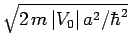is slightly less than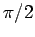. As the incident energy increases,, which is given by Eq. (1342), can reach the value. In this case,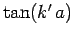becomes infinite, so we can no longer assume that the right-hand side of Eq. (1337) is small. In fact, it follows from Eq. (1337) that at the value of the incident energy whenthen we also have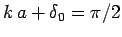, or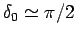(since we are assuming that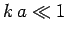). This implies that(1344)

Note that the cross-section now depends on the energy. Furthermore, the magnitude of the cross-section is much larger than that given in Eq. (1341) for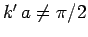(since).

The origin of this rather strange behaviour is quite simple. The condition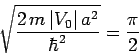(1345)

is equivalent to the condition that a spherical well of depthpossesses a bound state at zero energy. Thus, for a potential well which satisfies the above equation, the energy of the scattering system is essentially the same as the energy of the bound state. In this situation, an incident particle would like to form a bound state in the potential well. However, the bound state is not stable, since the system has a small positive energy. Nevertheless, this sort of resonance scattering is best understood as the capture of an incident particle to form a metastable bound state, and the subsequent decay of the bound state and release of the particle. The cross-section for resonance scattering is generally much larger than that for non-resonance scattering.

We have seen that there is a resonant effect when the phase-shift of the-wave takes the value. There is nothing special about the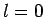partial wave, so it is reasonable to assume that there is a similar resonance when the phase-shift of the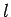th partial wave is. Suppose that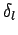attains the valueat the incident energy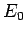, so that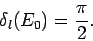(1346)

Let us expandin the vicinity of the resonant energy: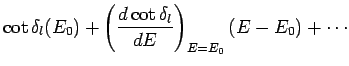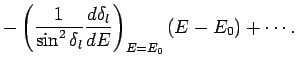(1347)

Defining(1348)

we obtain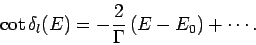(1349)

Recall, from Eq. (1308), that the contribution of theth partial wave to the scattering cross-section is(1350)

Thus,(1351)

This is the famous Breit-Wigner formula. The variation of the partial cross-section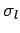with the incident energy has the form of a classical resonance curve. The quantity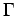is the width of the resonance (in energy). We can interpret the Breit-Wigner formula as describing the absorption of an incident particle to form a metastable state, of energy, and lifetime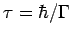.